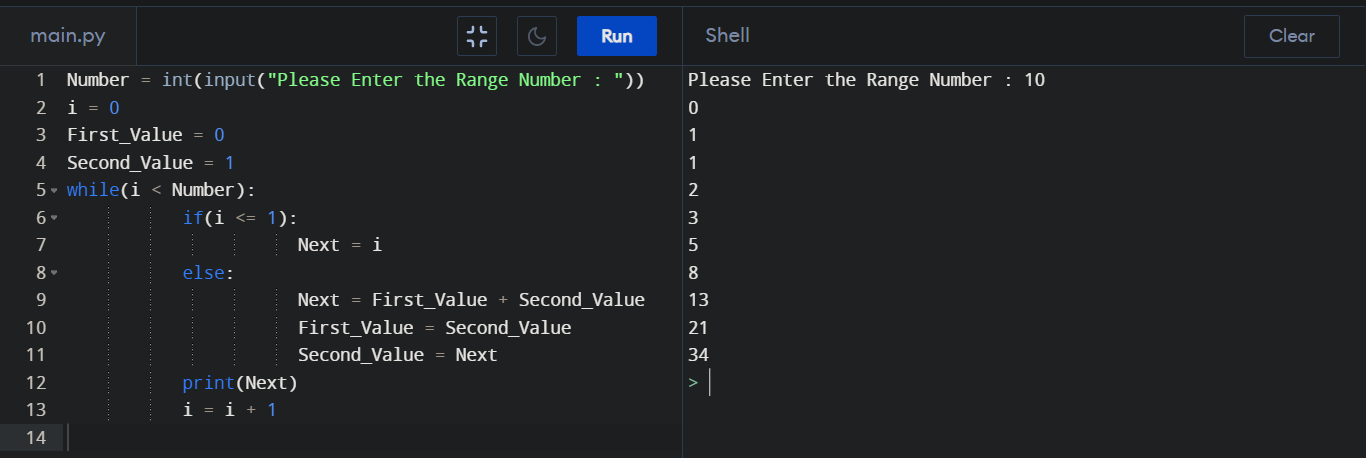Fibonacci Series program in Python

How to Write Fibonacci Series program? Fibonacci Series, or Fibonacci Numbers are the numbers displayed in the following sequence. Fibonacci Series = 0, 1, 1, 2, 3, 5, 8, 13, 21, 34 …

Python Fibonacci program :

Number = int(input("Please Enter the Range Number : "))
i = 0
First_Value = 0
Second_Value = 1
while(i < Number):
if(i <= 1):
Next = i
else:
Next = First_Value + Second_Value
First_Value = Second_Value
Second_Value = Next
print(Next)
i = i + 1

Output :